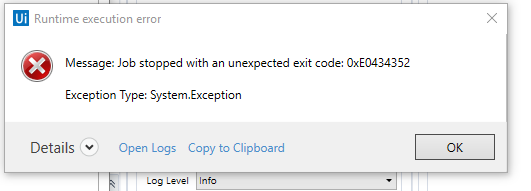# How to filter excel rows with more than 2 condition?

Hello, all

I need to filter rows with more than 2 condition.
Currently I can filter with 1 keyword(Array) condition but I’m struggling with filter more 2 condition.

Below each columns has to meet each Array variable. (FYI. with 1 condition, it’s working properly)
Column"Description" → St_Arr_keyword
(From row In Dt2_HSBC_001.Asenumerable
Where St_Arr_keyword.AsEnumerable.Where(Function(x) row(“Description”).ToString.Trim.Contains(x.trim)).Count>0
Select row).CopyToDatatable()

but I need to meet additional condition more than 2. For example,
Column"In" → St_Arr_keyword2
Column"Out" → St_Arr_keyword3

Thank you.

Hi @Dorothy_lee ,

You can use Filter Datatable activity for this.
https://docs.uipath.com/activities/docs/filter-data-table

1 Like

Than in below field(Yellow Remarked) , can I input ‘Array’ veriable?

@Dorothy_lee
Lets assume following

string arrays arrK1, arrK2

``````(From d in dtData.AsEnumerable
Let chk1 = arrK1.Any(Function (x) d("In").toString.Trim.Contains(x))
Let chk2 = arrK2.Any(Function (y) d("Out").toString.Trim.Contains(y))
Where {chk1,chk2}.All(Function (b) b)
Select r=d).CopyToDataTable
``````

in case of we do have many cols, then we can chek for some more generics. But lets start with above

1 Like

@ppr I’ve tried with above code and works properly ! thank you.
By the way, can I add one more value?
I’ve tried after adding chk3 but seem like not working.

(From d In ResDT.AsEnumerable
Let chk1 = St_Arr_keyword.Any(Function (x) d(“설명”).toString.Trim.Contains(x))
Let chk2 = St_Arr_keyword2.Any(Function (y) d(“입금 금액”).toString.Trim.Contains(y))

Let chk3 = St_Arr_keyword3.Any(Function (y) d(“Outplus”).toString.Trim.Contains(y))

Where {chk1,chk2,chk3}.All(Function (b) b)
Select r=d).CopyToDataTable()

could you please review what is wrong?

give a try on

``````(From d In dtData.AsEnumerable
Let chk1 = arrK1.Any(Function (k1) d("In").toString.Trim.Contains(k1))
Let chk2 = arrK2.Any(Function (k2) d("Out").toString.Trim.Contains(k2))
Let chk3 = arrK3.Any(Function (k3) d("Outplus").toString.Trim.Contains(k3))
Where {chk1,chk2, chk3}.All(Function (b) b)
Select r=d).CopyToDataTable
``````

try to have meanfull short variable names e.g.
St_Arr_keyword vs arrKeywordsSTR

avoid to use lambda arguments (x,y,d…) multiple times on different expressions. We are mor safe when these arguments are unique - e.g. the shift from x,y to k1,k2

1 Like

@ppr It works !! Thank you !
Sorry for too many Questions but can I convert it (Defiltered)?
I mean I’d like to reverse above condition to remain datatable(dtDate) except for data which contains above condition.

not sure if I got you. So let me summarize my understanding.

If a row has a match in all 3 keywords list then we dont want to get this row:

``````(From d In dtData.AsEnumerable
Let chk1 = arrK1.Any(Function (k1) d("In").toString.Trim.Contains(k1))
Let chk2 = arrK2.Any(Function (k2) d("Out").toString.Trim.Contains(k2))
Let chk3 = arrK3.Any(Function (k3) d("Outplus").toString.Trim.Contains(k3))
Where Not {chk1,chk2, chk3}.All(Function (b) b)
Select r=d).CopyToDataTable
``````

If a row has a match in 1 or more 3 keywords list then we dont want to get this row:

``````(From d In dtData.AsEnumerable
Let chk1 = arrK1.Any(Function (k1) d("In").toString.Trim.Contains(k1))
Let chk2 = arrK2.Any(Function (k2) d("Out").toString.Trim.Contains(k2))
Let chk3 = arrK3.Any(Function (k3) d("Outplus").toString.Trim.Contains(k3))
Where Not {chk1,chk2, chk3}.Any(Function (b) b)
Select r=d).CopyToDataTable
``````

Are you looking for this?

1 Like

@ppr Thank you so much!! This is what I meant !@ppr hello, with below code.
Can I get data except for null and “0”…?
since is has to many rows, when I try ‘Write Range’ it’s getting below error…

If(
(From d In ResDT_Ori.AsEnumerable
Let chk1 = St_Arr_keyword.Any(Function (k1) d(“Description”).toString.Trim.Contains(k1))
Let chk2 = St_Arr_keyword2.Any(Function (k2) d(“In”).toString.Trim.Contains(k2))
Where Not {chk1,chk2}.All(Function (b) b)
Select r=d).Count.Equals(0),
_
Nothing,
_
(From d In ResDT_Ori.AsEnumerable
Let chk1 = St_Arr_keyword.Any(Function (k1) d(“Description”).toString.Trim.Contains(k1))
Let chk2 = St_Arr_keyword2.Any(Function (k2) d(“In”).toString.Trim.Contains(k2))
Where Not {chk1,chk2}.All(Function (b) b)
Select r=d).CopyToDataTable
)This topic was automatically closed 3 days after the last reply. New replies are no longer allowed.